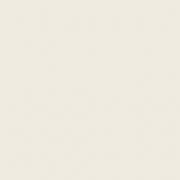1.## Librarian

Members

2

• ### Content count

657

2.## konstantin magnus

Members

1

• ### Content count

810

3.## Atom

Members

1

• ### Content count

3,266

4.Members

1

1,907

## Popular Content

Showing most liked content on 07/31/2020 in all areas

1. 1 point
http://www.cgchannel.com/2020/07/download-the-free-public-beta-of-cascadeur/ what is this sorcery...I'm not an animator but would an animator say this is a Maya killer ?
2. 1 point
3. 1 point
Thanks for the answer, very cool, didn't thought about that as I was blocked on just fixing the non-cutted faces and totally forgot that I could split all the faces like this instead of using the edge divide. Anyway, I found a solution to fix this, the code goes into a detail wrangle in a for loop over each bugged face : int pts[] = findattribval(0, "point", "bugged", 1); i[]@pts = pts; int p0_ = pts; int p1_ = pts; int in(int target; int array[]) { //iterate through list to find if a item is in or not int found = 0; foreach(int a; array) { if(target == a) found = 1; else found = 0; } return found; } void splitFace(int p0_, p1_, prim0) { // create neighbours pairs int p0[] = neighbours(0, p0_); int p1[] = neighbours(0, p1_); int x0_ = p0; int y0_ = p0; int x1_ = p1; int y1_ = p1; int x0[] = neighbours(0, x0_); int y0[] = neighbours(0, y0_); int x1[] = neighbours(0, x1_); int y1[] = neighbours(0, y1_); // create arrays int array1[], array2[]; //array 1 if(in(x0_, y1) == 1) { setcomp(array1, x0_, 0); setcomp(array1, y1_, 1); setcomp(array1, p1_, 2); setcomp(array1, p0_, 3); } if(in(x0_, x1) == 1) { setcomp(array1, x0_, 0); setcomp(array1, x1_, 1); setcomp(array1, p1_, 2); setcomp(array1, p0_, 3); } //array 2 if(in(y1_, y0) == 1) { setcomp(array2, p0_, 0); setcomp(array2, p1_, 1); setcomp(array2, y1_, 2); setcomp(array2, y0_, 3); } if(in(y0_, x1) == 1) { setcomp(array2, p0_, 0); setcomp(array2, p1_, 1); setcomp(array2, x1_, 2); setcomp(array2, y0_, 3); } setdetailattrib(0, "a1", array1, "set"); //debug setdetailattrib(0, "a2", array2, "set"); //debug // create polys removeprim(0, prim0, 0); addprim(0, "poly", reverse(array1)); addprim(0, "poly", reverse(array2)); } splitFace(p0_, p1_, 0);
4. 1 point
There's probably loads of ways to do this, but here's one: split_polys.hipnc
5. 1 point
https://zhuanlan.zhihu.com/p/97369808 have Fun
6. 1 point
To fill quadratic polygons with copies just take the square root of the intrinsic primitive area as point scale: int pt_add = addpoint(0, v@P); float area = primintrinsic(0, 'measuredarea', i@primnum); float scale = sqrt(area); setpointattrib(0, 'pscale', pt_add, scale, 'set'); removeprim(0, i@primnum, 1); KM_recursive_subd_001.hipnc
7. 1 point
Here is a basic setup. It uses a second loop to give each primitive a unique name. Inside the loop, the area for each primitive is stored as an attribute. After the loop, pscale is derived from the area. Use the ramp and the multiplier to dial in the sizes. ap_recursive_subd_001.hiplc
×
• Donations